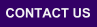Mplus
Thursday
June 08, 2023Mplus Mplus at a GlanceGeneral DescriptionMplus Programs Pricing Version History System Requirements Platforms
 Analyses/ResearchMplus Examples Papers References
 Search

How To Calculate The Power To Detect That A Parameter Is Different From Zero

1. Create a mean vector and covariance matrix for the hypothesized parameter values for the model of interest. This is done by specifying your model with all parameters fixed to the population values and asking for RESIDUAL. These population parameter values can come from past research or theory.
```TITLE:    Power calculation for a growth model
Step 1: Computing the population means
and covariance matrix

DATA:     FILE IS artific.dat;
TYPE IS MEANS COVARIANCE;
! Below is the data in artific.dat. The first
! row is the means.  The rest in the
! covariance matrix.
!   0 0 0 0
!   1
!   0 1
!   0 0 1
!   0 0 0 1

NOBSERVATIONS = 500;
! This number is arbitrary but should be no
! smaller than 500 to get sufficient
! precision.

VARIABLE: NAMES ARE y1-y4;

ANALYSIS: TYPE=MEANSTRUCTURE;

MODEL:    i BY y1-y4@1;
s BY y2@1 y3@2 y4@3;
[y1-y4@0];
[i@0 s@.2];

i@.5;
s@.1;
i WITH s@0;
y1-y4@.5;

OUTPUT:  STANDARDIZED RESIDUAL;
```
2. Analyze the mean and covariance matrix obtained from the residuals in the first step to check that a mistake was not made. The parameter values will be recovered if the mean and covariance matrix were created correctly.
```TITLE:    Power calculation for a growth model
Step 2: Analyzing the population means and
Covariance matrix to check that parameters
are recovered

DATA:     FILE IS pop.dat;
TYPE IS MEANS COVARIANCE;
NOBSERVATIONS = 500;
! Below is the data in pop.dat.
!  0 .2 .4 .6
!  1
!  .5 1.1
!  .5 .7 1.4
!  .5 .8 1.1 1.9

VARIABLE: NAMES ARE y1-y4;

ANALYSIS: TYPE=MEANSTRUCTURE;

MODEL:    i BY y1-y4@1;
s BY y2@1 y3@2 y4@3;

[y1-y4@0];

[i s];

OUTPUT:   STANDARDIZED RESIDUAL;
```
3. Analyze the mean and covariance matrix obtained from the first step with a model that misspecifies one parameter by fixing it to zero and specifies the number of observations for which power is wanted.
```TITLE:    Power calculation for a growth model
Step 3: Analyzing the population means and
Covariance matrix with a misspecified model

DATA:     FILE IS pop.dat;
TYPE IS MEANS COVARIANCE;
NOBSERVATIONS = 50;
! sample size for power

VARIABLE: NAMES ARE y1-y4;

ANALYSIS: TYPE=MEANSTRUCTURE;

MODEL:    i BY y1-y4@1;
s BY y2@1 y3@2 y4@3;

[y1-y4@0];

[i s@0];
!  The mean of the slope growth factor
!  is fixed to zero.

OUTPUT:   STANDARDIZED RESIDUAL;
```
4. Use the chi-square value of 9.286 from the third step as an approximate noncentrality parameter. Calculate the power to detect the misspecification using the SAS program below where the degrees of freedom are equal to one, the critical chi-square value for p of .05 is 3.841459, and the approximate noncentrality parameter is 9.286.
```DATA POWER;
DF=1; CRIT=3.841459;
LAMBDA=9.286;
POWER=(1-(PROBCHI(CRIT,DF,LAMBDA)));
RUN;
```
Chi-square values for other sample sizes can be calculated by using the fact that chi-square is equal to 2*sample size*F. Multiplying chi-square by the ratio of the new sample size to the sample size for the chi-square gives a chi-square for the new sample size that can be put into the SAS program above to obtain the power for that sample size. For example, the chi-square for sample size 100 is equal to 100/50*9.286 or 18.572. Following are the power results for this example.

 SAMPLE SIZE POWER 44 0.80 50 0.85 100 0.98 200 0.99